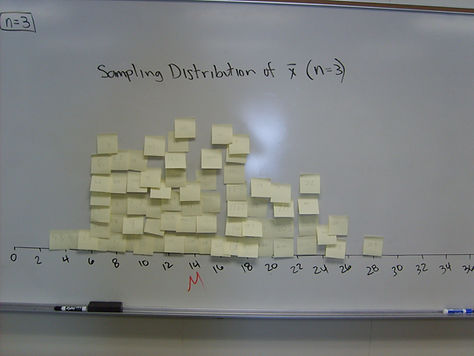top of page

## Chapter 7 - Day 11

##### Activity: Chapter 7 Review Sheet

Help keep all the ideas from Chapter 7 organized in this nice chart. Tell students that there is the “world of proportions” and the “world of means”. Some ideas are shared between the two worlds (10% condition) but many ideas are different (formulas for the sampling distributions, Normal check). We included some notes from Chapter 6 in case they encounter a review question on the Chapter 7 Test (evil laugh).

##### Two Optional Activities

Here are two activities that we really like, but did not have time for during Chapter 7.

##### Histogram:

In this Activity, students are trying to estimate the number of German Tanks available for World War II using a small sample of 5 tanks. Students should work in groups to come up with their own formula for calculating a good estimate. The formula they use to calculate their guess is a statistic. The rest of the activity investigates which statistic might be the best estimator. In the end, we want the statistic with low bias and low variability. You will need to prepare the “tanks” ahead of time. Print out the numbers, cut them out, and put them in ziplock bags. Each group will need a ziplock bag with the numbers 1 to 36.

##### 2. Age of Pennies Activity

To prepare for this activity, you have to gather a decent sized population of pennies. I usually ask each student to try and find 20 pennies to bring to class. In the Activity, students will be trying to estimate the average age of the population of pennies by taking a sample (using samples of size 3 and size 10). In the end, have students organize all of the pennies by age (population distribution). The amazing revelation is that the population distribution of age of pennies is skewed right (the older the penny the more likely it is to be lost in the couch), and the sampling distribution becomes closer to Normal as the sample size increases. Central Limit Theorem!

Population Distribution:Sampling Distribution (n = 3)Sampling Distribution (n = 10)bottom of page